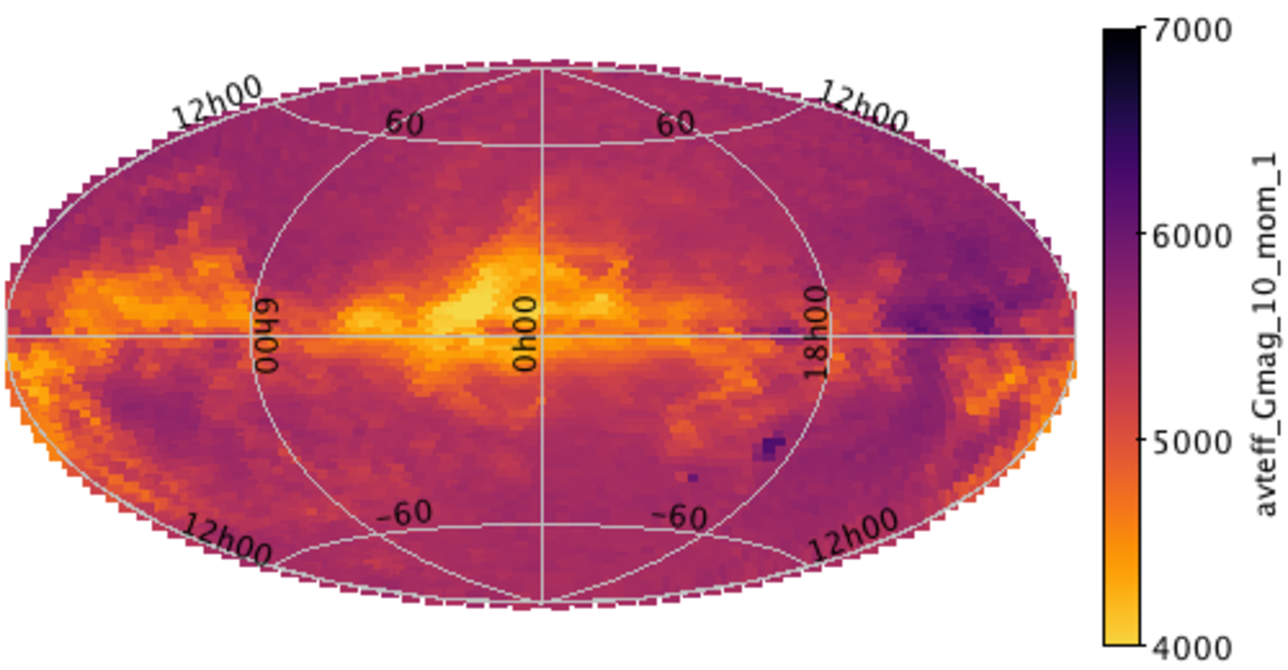# 10.3.5 Effective temperature distributions

Effective temperature (T${}_{\mathrm{eff}}$) distributions are computed for Gaia DR2 data and GOG18 simulations separately, then combined and inter-compared. Their absolute difference of the mean and standard deviations are computed. The maps are shown Figure 10.30 in two magnitude range $12 and $17 as examples. While in the model we do not expect any effect of extinction in the mean T${}_{\mathrm{eff}}$, it is clearly seen in the data. The only effect due to extinction in the model (seen in the figure) is the fact that when extinction becomes high at very low latitudes, the apparent magnitude of the stars is larger and there is a shift in the effective temperature. In the data, it seems that the correlations with extinction $A_{0}$ that is produced by the treatment in CU8 introduce a significant shift in the temperature. Figure 10.31 shows how the systematic difference in latitude varies with the latitude for two magnitude bins. It is notable that the systematic effect increases with magnitude as well. We conclude that in pre-DR2 release the temperatures at low latitudes (in a broad sense) suffer from a large systematic error which is a function of latitude and magnitude, which can reach 2000 K in the Galactic plane. This is most probably because temperatures have been derived assuming a null extinction.Figure 10.30: Mean effective temperature computed in HEALPix bins in 2 magnitude ranges (top: 13Figure 10.31: Difference in the mean temperature between Gaia DR2 data and GOG simulation, in HEALPix bins as a function of the latitude in two magnitude bins. Left: 12